## RS Aggarwal Class 7 Solutions Chapter 21 Collection and Organisation of Data (Mean, Median and Mode) Ex 21B

These Solutions are part of RS Aggarwal Solutions Class 7. Here we have given RS Aggarwal Solutions Class 7 Chapter 21 Collection and Organisation of Data Ex 21B.

Other Exercises

Question 1.
Solution:
(i) Arranging in ascending order.
2, 2, 3, 5, 7, 9, 9, 10, 11
Here number of terms = 9 which is odd
Median= $$\frac { n +1 }{ 2 }$$ = $$\frac { 9 + 1 }{ 2 }$$ th term
= 5th term = 7
Hence median = 7
(ii) Arranging in ascending order,
6, 8, 9, 15, 16, 18, 21, 22, 25
Here, number of terms (n) = 9 which is odd
Median= $$\frac { n +1 }{ 2 }$$ = $$\frac { 9 + 1 }{ 2 }$$ th term
= 5th term = 16
(iii) Arranging in ascending order,
6, 8, 9, 13, 15, 16, 18, 20, 21, 22, 25 Here number of terms (n) = 11 which is odd
Median= $$\frac { n +1 }{ 2 }$$ = $$\frac { 11 + 1 }{ 2 }$$ th term
= 6th term = 16

Question 2.
Solution:
(i) Arranging in ascending order,
9, 10, 17, 19, 21, 22, 32, 35
Here, number of terms = 8 which is even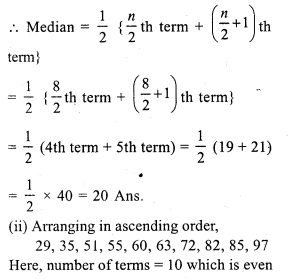Question 3.
Solution:
First 15 odd numbers are 1, 3, 5, 7, 9, 11, 13, 15, 17, 19, 21, 23, 25, 27, 29.
Here, number of terms (n) = 15 which is odd
Median = $$\frac { n + 1 }{ 2 }$$ = $$\frac { 15 + 1 }{ 2 }$$ th term
= 8th term = 15

Question 4.
Solution:
First 10 even numbers are 2, 4, 6, 8, 10, 12, 14, 16, 18, 20
Here, number of terms = 10 which is even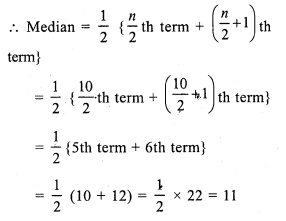Question 5.
Solution:
First 50 whole numbers
0, 1, 2, 3, 4, …, 49
Here, number of terms = 50, which is even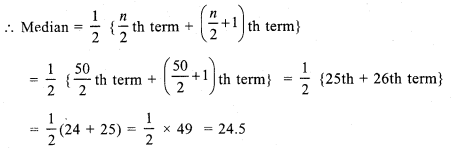Question 6.
Solution:
Arranging in ascending order,
17, 17, 19, 19, 20, 21, 22, 23, 24, 25, 26, 29, 31, 35, 40 .
Here, number of terms = 15 which is odd
Median = $$\frac { n + 1 }{ 2 }$$ th term = $$\frac { 15 + 1 }{ 2 }$$ = $$\frac { 16 }{ 2 }$$ th
= 8th term = 23

Question 7.
Solution:
Arranging is ascending order,
31, 34, 36, 37, 40, 43, 46, 50, 52, 53
Here, number of terms = 10, which is even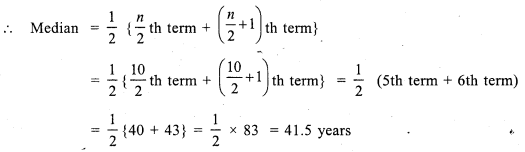Question 8.
Solution:
Preparing the cumulative frequency tableHere, number of terms (N) = 41, which is odd
Median = $$\frac { n + 1 }{ 2 }$$ th term
= $$\frac { 41 + 1 }{ 2 }$$ = $$\frac { 42 }{ 2 }$$ th = 21 th term = 50kg (value of 20 to 28 = 50)
Hence median = 50kg

Question 9.
Solution:
Arranging in order and preparing the cumulative frequency table.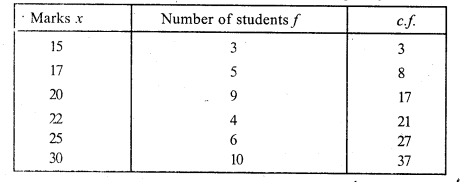Here, number of terms (N) = 37 which is odd.
Median = $$\frac { n + 1 }{ 2 }$$ th term
= $$\frac { 37 + 1 }{ 2 }$$ = $$\frac { 38 }{ 2 }$$ th term
= 19th term = 22 (Value of 18 to 21 = 22)
Hence median = 22

Question 10.
Solution:
Arranging in order and then preparing its cumulative frequency table :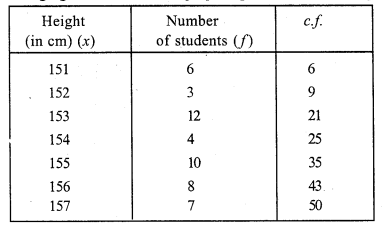Here, number of terms (N) = 50, which is evenHence median = 154.5 cm

Hope given RS Aggarwal Solutions Class 7 Chapter 21 Collection and Organisation of Data Ex 21B are helpful to complete your math homework.

If you have any doubts, please comment below. Learn Insta try to provide online math tutoring for you.